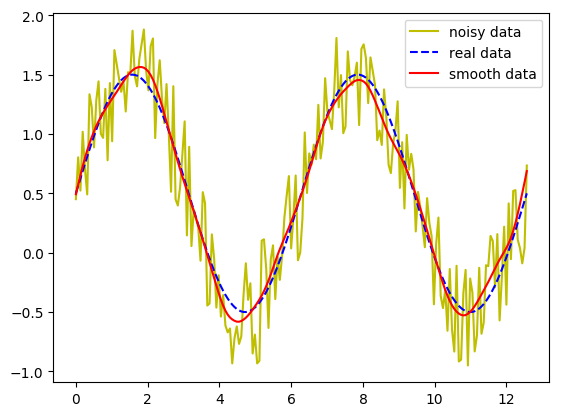# How to smoothen noisy data

The data points that I obtain in my core flooding experiments are very noisy, due to the fluctuations in the back pressure valve and the unsable nature of foam. I use smooth function of Matlab to make the data smoother mostly for a better visualization of the results. I missed this function in Julia, until I found this Python code that I could call from Julia thanks to PyCall package. The code is quite simple. It mirrors the data at the begining and the end of the data array, and uses scipy's convolution function, which is also available in Julia. For the window functions, I used the DSP package. This is the smooth function:

In :
function smooth(y, win_len=11, win_method=2)
# This function requires the DSP package to be installed
# 1: flat
# 2: hanning
# 3: hamming ...
if win_len%2==0
win_len+=1 # only use odd numbers
end
if win_method == 1
w=ones(win_len)
elseif win_method==2
w=DSP.hanning(win_len)
elseif win_method==3
w=DSP.hamming(win_len)
end

if win_len<3
return y
elseif length(y)<win_len
return y
else
y_new = [2*y-flipdim(y[1:win_len],1); y[:]; 2*y[end]-flipdim(y[end-win_len:end],1)]
y_smooth = conv(y_new, w/sum(w))
ind = floor(Int, 1.5*win_len)
return y_smooth[1+ind:end-ind-1]
end

end # end of function

Out:
smooth (generic function with 3 methods)

To use this package, we need to have the DSP package installed. Let's try the function here:

In :
using DSP, PyPlot;

In :
x=linspace(0,4π,200)
y_real=0.5+sin.(x)
y_noisy=sin.(x)+rand(size(x))
y_smooth=smooth(y_noisy, 25)
plot(x,y_noisy, "y", x, y_real, "b--", x, y_smooth, "r")
legend(["noisy data", "real data", "smooth data"]);Due to laziness, I've only included three window functions. You can add more functions if you like!

### Update:¶

flipud function is deprecated in Julia 0.5 and 0.6. I have replaced it with flipdim function in the code. I also add an Int argument to the floor command to force it to return an integer index. Now the code works in Julia 0.6 as well.